Next: Iterative methods Up: Solutions Previous: Solutions

## Algebraic methods

The algebraic solution of equation (2) exists only for a restricted class of cases. The joint angles could be expressed using the end effector position. The number of nonlinear equations increases with the DOFs (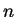DOFs meanequations). Each joint angle - one by one - could be solved by the system ofequations [Chi96]: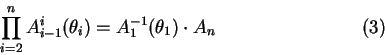The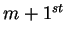joint angle is expressed using the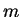previous angles. The last joint angle is expressed only by the end effector position and orientation.

The forward kinematics solution for the end effector position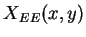in 2 DOF structure could be expressed as follows: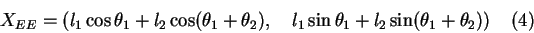Thus, by applying elementary trigonometry the inverse solution is: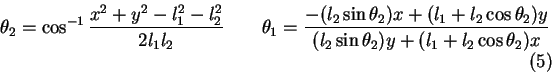As the DOFs for most of cases are higher, the state vector is not analytically expressible using such a trivial way. Therefore more sophisticated approaches are necessary.

Lukas Barinka 2002-03-21